# Magic Squares

##### Age 14 to 18

Published 1998 Revised 2018

A basic introduction to magic squares can be found here .

Magic squares have intrigued people for thousands of years and in ancient times they were thought to be connected with the supernatural and hence, magical. Today, we might still think of them as being magical, for the sum of each row, column and diagonal is a constant, the magic constant. The squares intrigued me when I found that their construction was far from easy. For the simple 3x3, that is order 3 magic square, trial and improvement quickly does the job; but for higher than order 4 magic squares a method is necessary. The problem of construction is twofold. An algorithm which works for odd order squares will not work for even order squares without the further addition of another algorithm. At least, I know of no method which will work for both odd and even orders, other than trial and improvement computer programs. For the purposes of this article, I will be considering only magic squares that are constructed using consecutive integers from 1 to n 2 , where n is the number of integers on one side of the square.

Odd magic squares are fairly easily constructed using the either the Siamese (sometimes called de la Loubere's, or the Staircase method), the Lozenge, or the de Meziriac's methods.
De Meziriac's method can be found on page 76 in the book "Mathematical Games and Puzzles'', by Trevor Rice and published by B.T Batsford Limited, London. Another way, which I prefer (but then that was the way I learned to construct odd order squares), is the extended Pyramid method or diagonals. This method consists of three steps:

1. Draw a pyramid on each side of the magic square. The pyramid should have two less squares on its base than the number of squares on the side of the magic square. This creates a square standing on a vertex.
2. Sequentially place the numbers 1 to n 2 of the n x n magic square in the diagonals as shown in Figures 1 and 2.
3. Relocate any number not in the n x n square (that appears in the pyramids you added) to the opposite hole inside the square (shaded).

#### Figure 1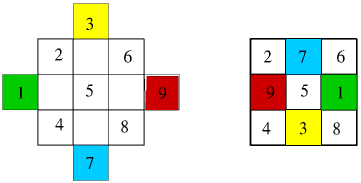The same Pyramid method can be used for any odd order magic square as shown below for the 5x5 square in Figure 2.

#### Figure 2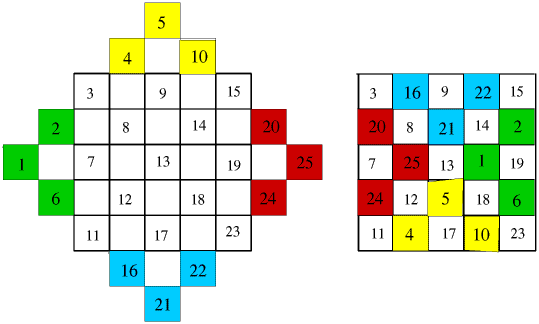We can use some properties of magic squares to construct more squares from the manufactured squares above; e.g.

1. A magic square will remain magic if any number is added to every number of a magic square.
2. A magic square will remain magic if any number multiplies every number of a magic square.
3. A magic square will remain magic if two rows, or columns, equidistant from the centre are interchanged.
4. An even order magic square ( n x n where n is even) will remain magic if the quadrants are interchanged.
5. An odd order magic square will remain magic if the partial quadrants and the row is interchanged.

This will be the subject of the next article published in September.

Constructing the even order magic squares does present more of a challenge.
All the methods I have seen in the literature are rather complicated, in that they require the use of two or more algorithms. There are claims for a simple method for the construction of even order magic squares, but I have yet to find such a method. However, the following method, which I have developed, uses but one algorithm and will work for any even sided square. I call it the "paired exchange method". The theory behind the paired method is fairly straightforward.

Consider the first and last column of a n x n magic square where n is even. Starting by placing the integers in order across the rows of the square (see figure 3), the difference between the first and last number in any row will be n - 1. Since there are n rows in the square, there will be a total difference of n ( n -1) between the first and last column of the square. To balance the total for the first and last columns we must exchange pairs of numbers between the first and last columns and each exchanged pair must be from the same row, so as not to change the sum total of the row. How many times must we exchange pairs to equalise the columns? When a pair is exchanged in a row the difference between the columns changes by 2( n -1). If t is the number of times pairs must be exchanged, then

 t [2( n - 1)] = n ( n - 1), 2 t = n , and t = n /2.

A similar argument can be made for the 2 nd and the next to last columns, since the only change in the above formulae will be to substitute ( n - 3) for ( n - 1). The resulting t stays the same. In like manner, all columns paired from the centre line of the square can be made to be equal, and since the numbers in the original square are consecutive integers, all the columns will be equal to the magic constant for the n by n magic square. Of course, columns are just rows seen from a different viewpoint, hence in a like manner all rows can be made to equal the magic constant. Now let's look at a few examples.

Consider the 4 by 4 magic square. t is now equal to 2. The choice of pairs to exchange is limited if we want the sum of the numbers on the diagonals to equal the magic constant. The pairs must be on the diagonals. By reflecting in the centre lines x and then y we achieve the same as a single reflection in the lines y = x and y = - x . Or to state it another way, we exchange the numbers with their opposite numbers equidistant from the centre along the diagonals (see figure 3). We have exchanged two pairs in each row and column of the square and the results is a magic square.

#### Figure 3The 6 by 6 magic square is constructed in the same manner, but here t = 3, hence we have much more freedom in our choice of pairs for each column and row. We exchange all the pairs on the diagonal, which is equivalent to exchanging two pairs from each pair of rows and columns, so we must now exchange one more pair from each pair of rows and columns to equalise them. One such choice in the construction of a 6 by 6 magic square is shown in figures 4, 5 and 6 below.

#### Figure 4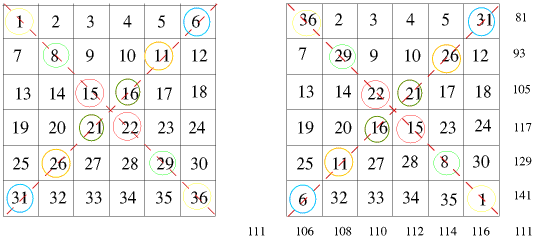Exchange the pairs in the diagonals.

Here we equalise the rows before the columns, the opposite order to that discussed in the text. It can be done in any order.

#### Figure 5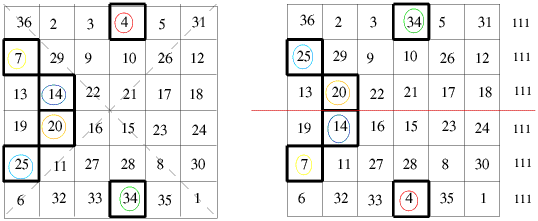Exchange the pairs in the columns.

#### Figure 6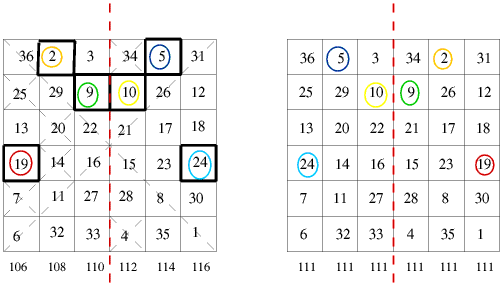Exchange the pairs in the rows.

How many other choices we have is possible to calculate, but I will leave that for another time. In the meantime try making a 7 by 7 magic square, or a 8 by 8, or a 10 by 10, or ....

The next article in the series is Magic Squares II.